## Free Fall - Complete Toolkit

### Objectives

1. To know the meaning of free fall and the conditions under which it occurs.
2. To know the value for the acceleration of gravity (g) and to relate this value to velocity-time information.
3. o relate the motion of a free-falling object to the graphical descriptions of its motion.
4. To use equations to calculate how fast and how far a free-falling object will move in a given amount of time.
5. To understand that the acceleration of gravity is independent of the mass of the free-falling object.

### Readings from The Physics Classroom Tutorial

1. The Physics Classroom Tutorial, 1-D Kinematics Chapter, Lesson 5
http://www.physicsclassroom.com/class/1DKin/Lesson-5/Introduction

### Interactive Simulations

1. Concord Consortium:  Was Galileo Right?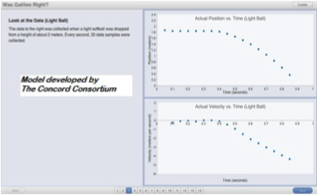This HTML5 graphing activity runs directly in a web browser to bring students a rich digital investigation of the effects of gravity on light and heavy objects. First, students use a graph- sketching tool to predict the P/T and V/T graphs for a light ball falling 2 meters to the ground. Next, they repeat the prediction for a heavy ball. Graphs are then automatically generated to show data based on actual timed trials. As students compare accurate experimental results to their predictions, they perform scaffolded calculations to determine slope. Expected outcomes: Students discover that 1) heavy and light objects fall at the same rate of acceleration, and 2) acceleration can be calculated from data in a Velocity vs. Time graph. Appropriate for beginners with little prior knowledge of free fall.

2. Open Source Physics:  Free Fall Model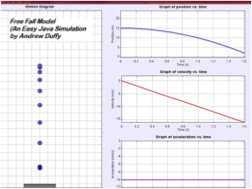This very simple Java simulation lets students explore the motion of an object in free fall. You can set the initial height (0-20m), set an initial velocity from -20 to 20 m/s, and change the rate of gravitational acceleration from zero to 20 m/s/s. You can also launch the ball upward from any point on the line of motion. The free fall is displayed as a motion diagram, while graphs are simultaneously displayed showing Position vs. Time, Velocity vs. Time, and Acceleration vs. Time.

3. Open Source Physics:  Ceiling Bounce Model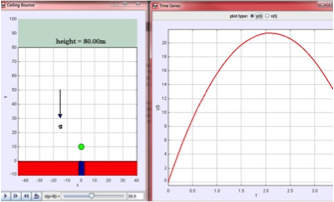This simulation by Wolfgang Christian shows a ball launched by a spring-gun in a building with a very high ceiling. The student's task is to calculate an initial velocity so that the ball barely touches the 80-foot ceiling. Students can test their answers by setting the initial velocity on the simulation, then watch the ball's path. Graphs of position vs. time or velocity vs. time are automatically generated. This model is especially helpful for visualizing the relationship between the one-dimensional motion of this example and its graph, as it displays the ball continuously bouncing at constant velocity in a straight line from floor to ceiling. There is no horizontal displacement.

4. Open Source Physics:  Free Fall RideThis EJS (Easy Java Simulation) model combines the acceleration of gravity with the acceleration of an elevator moving up or down. First, set the height and time, then watch the simulated motion. A graph of height vs. time is displayed alongside. You can drag points on the height vs. time graph to customize the elevator ride. The authors have given this sim extra kick by including a G-force vs. Time graph, which shows the apparent weight the rider feels. With strong enough downward acceleration, the rider will experience negative g's (effective weightlessness).

5. Open Source Physics:  Free Fall Air Resistance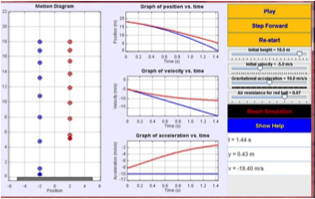In teaching free fall, it's good to be prepared for student confusion over why, for example, the free falling bowling ball hits the ground faster than the free falling leaf. We recommend two resources to introduce the idea of air resistance (which will be covered in more depth within Newton's Laws).  First, we recommend the Free Fall Air Resistance model linked above. Students can compare the motion of 2 free falling balls -- one falling under the influence of gravity alone and the other experiencing air resistance. The model generates simultaneous position, velocity, and acceleration graphs for BOTH falling objects, plus side-by-side dot diagrams. After exploring this model, we recommend showing the historic file footage (see Video section below) of the Apollo 15 Hammer and Feather Drop.

### Video and Animations

1. Apollo 15 Hammer and Feather Video 1-minute videoAt the end of the Apollo 15 moonwalk, Commander David Scott performed a live demonstration for TV cameras. He held out a geologic hammer and a feather and dropped them at the same time. Because the moon has no atmosphere (thus no air resistance), the feather and hammer both hit the ground at the same time. As Galileo had concluded hundreds of years before - all objects released together fall at the same rate of gravitational acceleration, regardless of mass. Caveat: This video is in the public domain, so there are many YouTube versions of it. This one was the highest quality we could locate.  NASA also has a library archive for all video clips associated with Apollo 15, but the format is limited to QuickTime or MPG. (1 minute, 20 seconds)

2. Cornell College: Slow Motion Water Drops Video DemonstrationThis 3-minute video was created by Cornell College physics professor Derin Sherman to illustrate free fall by pumping green-dyed water droplets out of a reciprocating pump, then illuminating them with a strobe light.  To the human eye, the droplets appear to fall in slow motion (but it's really an illusion due to the periodic nature of the pump). You can easily see the acceleration due to gravity. The resolution is high enough to perform video analysis. The author also gives directions to duplicate the demo in your own class, including building your own strobe light at a fraction of the cost of an industrial model.    If you're fascinated by strobe photography (as we are), you'll also be delighted by this 20-minute video of the life and contributions of "Doc" Edgerton, the iconic MIT professor of electrical engineering who transformed strobe photography.

3. Veritasium:  Misconceptions About Falling Objects 3.5-minute video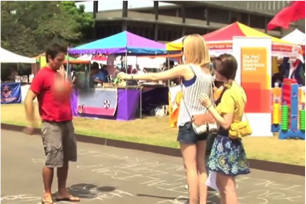Watch this all the way through! At first, it seems too simplistic for high school physics. But it packs a powerful punch at the end to prompt deep thinking about the difference between gravitational force and gravitational acceleration. Veritasium videos were created by Derek Muller, who holds a doctorate in PER (Physics Education Research) from the University of Sydney. His YouTube channel has attracted millions of followers -- partly because the science is reliably accurate, but mostly because they're fun to watch.

4. Rutgers University Physics Department: Galileo’s Pisa Experiment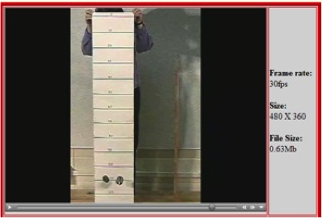This learning cycle features three video experiments, organized sequentially for introducing concepts of free fall to introductory physics students. Each video includes learning goal, prior knowledge required, and discussion questions. The first video shows simultaneous drop of two objects with different masses. The second shows non-simultaneous drop of two objects. The third asks students to quantitatively compare the two situations.  Motion can be stepped to allow video analysis, and each video can be individually downloaded for flexibility of use.

5. MIT Tech TV:  Strobe of a Falling Ball One-minute video demonstration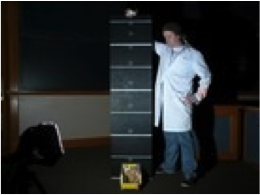In this short video clip, a ball is dropped in front of a meter stick and lit by a strobe light. A long exposure photograph captures the position of the ball at each evenly spaced flash of light. The acceleration of the ball can then be measured from the photo. Note: If your school doesn't allow direct online video viewing, this resource can also be downloaded in an MP4 version.

6. Free Fall Lab The Physics Classroom's YouTube Channel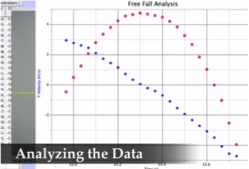The Physics Classroom demonstrates the use of video analysis to analyze a free falling object. The vertical acceleration and other trajectory characteristics are determined from analysis of the graphs.

7. Physlet Physics:  Cargo Cut from a Hot Air Balloon Animation-Based ProblemThis animated problem from Physlet Physics presents the scenario of cargo free-falling from a position at rest. Given the coordinates of the cargo, students are asked to calculate the velocity of the cargo before it was cut loose to free fall, calculate the maximum height reached by the cargo during its ascent, and figure out instantaneous velocity of the cargo at the instant before it hits the ground.

### Labs and Investigations

1. The Physics Classroom, The Laboratory, Free Fall
Students use a motion detector to determine the acceleration of a falling object.

2. The Physics Classroom, The Laboratory, Dune Buggy Challenge
This challenging lab project requires that students combine information about free falling motion and linear motion in order to calculate when a marble must be released in order for it to land is a toy car that is moving along the table below.

### Minds On Physics Internet Modules:

The Minds On Physics Internet Modules are a collection of interactive questioning modules that target a student’s conceptual understanding. Each question is accompanied by detailed help that addresses the various components of the question.
1. Newton's Laws, Ass’t NL10 -  Free Fall Acceleration

### Concept Building Exercises:

1. The Curriculum Corner, 1-D Kinematics, Free Fall

### Problem-Solving Exercises:

1. The Calculator Pad, 1-D Kinematics, Problems #25 - #28

### Science Reasoning Activities:

1. Science Reasoning Center, 1-D Kinematics, Kinematics

### Real Life Connections:

1. Problem-Based Learning:  Bungee Jumping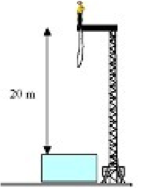In this PBL scenario, students are given a rough design for a bungee jump from a 20-meter tower. They work cooperatively to figure out the parameters for a safe jump. This problem requires them to apply concepts of gravitational acceleration and conservation of energy. Note: "Problem Based Learning" is not to be confused with "Project Based Learning".  In Problem-Based Learning, students are presented with real scenarios that mirror problems encountered by practicing scientists & engineers. The problems are crafted to provide both relevant and irrelevant information. Students will be required to fill in gaps by doing their own information discovery, distinguish useful from irrelevant data, and then work on teams to apply physics/math in finding solutions.

### Common Misconception:

1. Acceleration of Gravity and Mass

Many students believe that a more massive object will free fall faster than a less massive object. While this dependence upon mass is relevant to situations in which objects fall under the influence of air resistance, it does not apply to free-falling objects. All objects, regardless of mass, will free fall at a rate of 9.8 m/s/s.

### Elsewhere on the Web:

1. Using Direct Measurement Videos to Teach Physics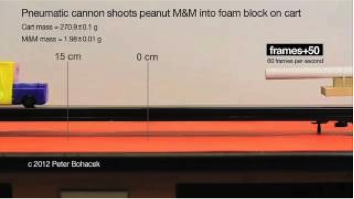This resource, part of the SERC Project (Science Education Resource Center), describes how to implement direct measurement videos for active learning in introductory physics classrooms. These high-resolution short videos feature tools that allow students to easily analyze physical situations (tools include grids, rulers, frame-counters and other screen overlays for making precise measurements). This material includes best practices for using Direct Measurement Videos, a library of videos, example class activities, and a set of model lessons with teaching tips.

### Standards:

A. Next Generation Science Standards (NGSS)

Performance Expectations
• HS-PS2-1:     Analyze data to support the claim that Newton’s second law of motion describes the mathematical relationship among the new force on a macroscopic object, its mass, and its acceleration.  (Clarification statement:  Examples of data could include tables or graphs of position or velocity as a function of time for objects subject to a net unbalanced force, such as a falling object.)

Disciplinary Core Ideas

Motion and Stability-Forces and Interactions
• MS-PS2.B.iii     Forces that act at a distance (electric, magnetic, and gravitational) can be explained by fields that extend through space and can be mapped by their effect on a test object (a charged object or a ball, respectively).   Limited alignment
• HS-PS2.A.i       Newton’s second law accurately predicts changes in the motion of macroscopic objects.

Science and Engineering Practices

Practice #3: Planning and Carrying Out Investigations
• Plan and conduct an investigation individually and collaboratively to produce data to serve as the basis for evidence … and consider limitations on the precision of the data

Practice #5: Using Mathematics and Computational Thinking
• Create and/or revise a computational model or simulation of a phenomenon, designed device, process, or system.
• Use mathematical and/or algorithmic representations of phenomena or design solutions to describe and/or support claims and explanations.
• Use a computational representation of phenomena to describe and/or support claims and/or explanations

B. Common Core Standards for Mathematics – Grades 9-12

Standards for Mathematical Practice:
• MP.1    Make sense of problems and persevere in solving them.
• MP.2    Reason abstractly and quantitatively

High School Algebra – Creating Equations
• A-CED.4   Rearrange formulas to highlight a quantity of interest, using the same reasoning as in solving equations.

High School – Functions
• F-IF.4     For a function that models a relationship between two quantities, interpret key features of graphs and tables in terms of the quantities, and sketch graphs showing key features given a verbal description of the relationship.
• F-IF.8.b     Use the properties of exponents to interpret expressions for exponential functions.

High School-Vector and Matrix Quantities
• N-VM.1     Recognize vector quantities as having both magnitude and direction. Represent vector quantities by directed line segments, and use appropriate symbols for vectors and their magnitudes.
• N-VM.3     Solve problems involving velocity and other quantities that can be represented by vectors.

C. Common Core Standards for English/Language Arts (ELA) – Grades 9-12
Key Ideas and Details: High School
• RST.11-12.2     Determine the central ideas or conclusions of a text; summarize complex concepts, processes, or information presented in a text by paraphrasing them in simpler but still accurate terms.
• RST.11-12.3     Follow precisely a complex multistep procedure when carrying out experiments, taking measurements, or performing technical tasks; analyze the specific results based on explanations in the text.

Craft and Structure – High School
• RST.11-12.4     Determine the meaning of symbols, key terms, and other domain-specific words and phrases as they are used in a specific scientific or technical context relevant to grades 11—12 texts and topics.

Integration of Knowledge and Ideas – High School
• RST.11-12.9     Synthesize information from a range of sources (e.g., texts, experiments, simulations) into a coherent understanding of a process, phenomenon, or concept, resolving conflicting information when possible.

Range of Reading and Level of Text Complexity – High School
• RST.11-12.10  -- By the end of grade 12, read and comprehend science/technical texts in the grades 11-CCR text complexity band independently and proficiently.

D. College Ready Physics Standards (Heller and Stewart)

Essential Knowledge
Newton’s Laws of Motion
• High School H.3.1.5     When the change in an object’s instantaneous velocity is the same in each successive unit time interval, the object has constant acceleration. For straight-line motion, constant acceleration can be represented by and calculated from a linear instantaneous velocity vs. time graph, a motion diagram, and the mathematical expression [a = (vf - vi)/(tf - ti)]. The sign (+ or -) of the constant acceleration indicates the direction of the change-of-velocity vector. A negative sign does not necessarily mean that the object is traveling in the negative direction or that it is slowing down. (Boundary: The term “deceleration” should be avoided because students tend to associate a negative sign of acceleration only with slowing down.)
• High School H.3.1.7      When the acceleration is constant, the magnitude of the average velocity during a time interval is one-half of the sum of the initial and final instantaneous velocities  [v = (vf + vi)/2].

Gravitational Interaction and Forces
• High School H.3.4.4     When people are in free fall, they feel “weightless” because people do not feel the extremely small gravitational force on each atom in their bodies. When standing, people feel the (normal) force of the ground pushing upwards on their feet, which produces the sensation of weight.
• High School H.3.4.3     When an object’s distance from Earth’s surface is small compared to Earth’s radius, then a simplifying assumption is that the gravitational force on an object depends only on the mass of the object. In this case, objects fall with approximately the same acceleration: 9.8 m/sec/sec.

• Explain what is “constant” when an object is moving with a constant acceleration, the two ways in which an object that has a positive constant acceleration can be moving (speeding up or slowing down) and the two ways in which an object that has a negative constant acceleration can be moving (speeding up or slowing down). Justify the explanations by constructing sketches of motion diagrams and using the shape of instantaneous velocity versus time graphs.
• Translate between different representations of the motion of objects: verbal and/or written descriptions, motion diagrams, data tables, graphical representations (position vs. time graphs and instantaneous velocity vs. time graphs) and mathematical representations.
• Evaluate the evidence for claims about the velocity or acceleration of objects in different experimental problems, using the criteria: (a) appropriate match of the evidence to the question or prediction; (b) adequate precision and accuracy; (c) correctness or data analysis and representation procedures (e.g., error bars on graphs for best estimate of slopes; and (d) the investigation was replicated (by other groups or classes).   Partial alignment

Learning Outcomes:  Gravitational Interactions and Forces-Grades 9-12
• Explain why all objects near Earth’s surface fall with approximately the same acceleration, despite having different masses and weights. Justify by using the universal law of gravitation and Newton’s Second Law.